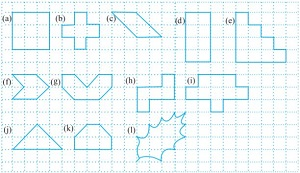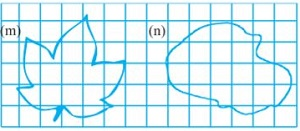### Mensuration-SolutionsEx-10.2

CBSE Class –VI Mathematics
NCERT Solutions
Chaper 10 Mensuration (Ex. 10.2)

Question 1. Find the areas of the following figures by counting squares:Answer: (a) Number of filled square = 9
Area covered by squares = 9 x 1 = 9 sq. units
(b) Number of filled squares = 5
Area covered by filled squares = 5 x 1 = 5 sq. units
(c) Number of full filled squares = 2
Number of half filled squares = 4
Area covered by full filled squares = 2 x 1 = 2 sq. units
And Area covered by half filled squares = 1/2 x 4 = 2 sq. units
Total area = 2 + 2 = 4 sq. units
(d) Number of filled squares = 8
Area covered by filled squares = 8 x 1 = 8 sq. units
(e) Number of filled squares = 10
Area covered by filled squares = 10 x 1 = 10 sq. units
(f) Number of full filled squares = 2
Number of half filled squares = 4
Area covered by full filled squares = 2 x 1 = 2 sq. units
And Area covered by half filled squares = 1/2 x 4 = 2 sq. units
Total area = 2 + 2 = 4 sq. units
(g) Number of full filled squares = 4
Number of half filled squares = 4
Area covered by full filled squares = 4 x 1 = 4 sq. units
And Area covered by half filled squares = 1/2 x 4 = 2 sq. units
Total area = 4 + 2 = 6 sq. units
(h) Number of filled squares = 5
Area covered by filled squares = 5 x 1 = 5 sq. units
(i) Number of filled squares = 9
Area covered by filled squares = 9 x 1 = 9 sq. units
(j) Number of full filled squares = 2
Number of half filled squares = 4
Area covered by full filled squares = 2 x 1 = 2 sq. units
And Area covered by half filled squares = 1/2 x 4 = 2 sq. units
Total area = 2 + 2 = 4 sq. units
(k) Number of full filled squares = 4
Number of half filled squares = 2
Area covered by full filled squares = 4 x 1 = 4 sq. units
And Area covered by half filled squares = 1/2 x 2 = 1 sq. units
Total area = 4 + 1 = 5 sq. units
(l) Number of full filled squares = 3
Number of half filled squares = 10
Area covered by full filled squares = 3 x 1 = 3 sq. units
And Area covered by half filled squares = 1/2 x 10 = 5 sq. units
Total area = 3 + 5 = 8 sq. units
(m) Number of full filled squares = 7
Number of half filled squares = 14
Area covered by full filled squares = 7 x 1 = 7 sq. units
And Area covered by half filled squares = 1/2 x 14 = 7 sq. units
Total area = 7 + 7 = 14 sq. units
(n) Number of full filled squares = 10
Number of half filled squares = 16
Area covered by full filled squares = 10 x 1 = 10 sq. units
And Area covered by half filled squares = 1/2 x 16 = 8 sq. units
Total area = 10 + 8 = 18 sq. units